How to Round Numbers in Excel Using Various Excel Rounding Formulas/Functions - Compute Expert

# How to Round Numbers in Excel Using Various Excel Rounding Formulas/Functions

Home >> Excel Tutorials from Compute Expert >> Excel Calculations >> How to Round Numbers in Excel Using Various Excel Rounding Formulas/Functions

In this tutorial, you will learn how to round numbers in excel using various rounding functions available in excel. You will also learn how to round numbers using excel features other than functions.

When we process our numbers in excel, mastering the number rounding methods in the software is important. This is especially true if we often work with decimal numbers. Excel has many rounding formulas that you can use depending on the kind of result you want to get.

Curious what are those rounding formulas, how to use them, and what kind of result you can expect from them? Want to know the other features you can use to round numbers in excel besides formulas? Read this excel number rounding tutorial from Compute Expert until its last part!

Disclaimer: This post may contain affiliate links from which we earn commission from qualifying purchases/actions at no additional cost for you. Learn more

## List of Excel Rounding Formulas: a Brief Summary

As mentioned before, there are many number rounding formulas you can use in excel. Each of them has its own uniqueness in terms of usefulness and usage method.

Below is a table that summarizes 12 rounding formulas available in excel and their functions. The list may not be exhaustive but it should be pretty complete and contains the formulas you most likely use. In the next parts of this tutorial, we will discuss the basics and how to use each of these formulas.

FormulaUse it to...
ROUNDround decimals normally (round 0-4 down and round 5-9 up)
ROUNDUPround up decimals
ROUNDDOWNround down decimals
MROUNDround a number to a particular multiple normally (round bottom half of the multiple down and round upper half of the multiple up)
CEILING/CEILING.MATHround up a number to a particular multiple
FLOOR/FLOOR.MATHround down a number to a particular multiple
INTround down a number with decimals to the nearest integer
TRUNCremove/truncate decimals from a number
EVENround up a number with decimals to the nearest even integer
ODDround up a number with decimals to the nearest odd integer
DOLLARround a number with decimals normally and convert the number to currency<
FIXEDround a number with decimals normally and convert the number to a formatted number text

Let’s begin discussing these formulas implementation with the most standard excel rounding formula, ROUND!

## How to Round Decimals Normally in Excel: ROUND

Want to round those decimals in your number normally? Then, you should use ROUND.

ROUND will round 0 to 4 down and 5 to 9 up like a normal rounding process. The formula writing form of ROUND is like what you can see below.

= ROUND ( number_to_round , number_of_decimals_in_result )

Just input the number you want to round and the decimals you need in your rounding result to ROUND. You will immediately get the rounding result you want from the formula!

Here is the implementation example of ROUND in excel.As you can see, ROUND will round our number to the number of decimals we input.

If we input the same or more number of decimals than what our number has currently, ROUND won’t do anything. If we input 0 for the number of decimals, then ROUND will round our number to the nearest integer.

If we input a negative number for that, then ROUND will round our number to the nearest powered 10 multiple. The power level of the 10 multiple depends on the negative number value we input (-1 means 10 powered by 1 or 10, -2 means 10 powered by 2 or 100, and so on).

## How to Round Up Decimals in Excel: ROUNDUP

What if we want to round up our number instead of round it normally? This question calls for the ROUNDUP implementation.

The formula writing of ROUNDUP is similar to the ROUND formula we just discussed.

= ROUNDUP ( number_to_round_up , number_of_decimals_in_result )

Only the function is different between these two formulas. ROUNDUP will round up our decimals while ROUND will round them normally.

You can see the rounding-up results from ROUNDUP in the screenshot below.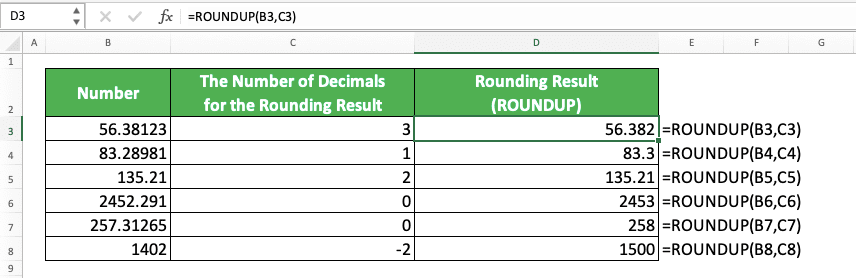As you can see there, ROUNDUP will also round up 0-4 in your decimals (56.38123 to 56.382 instead of 56.381, 2452.291 to 2453 instead of 2452, etc). Its treatment to the same or more, zero, or a negative number of decimals input is the same as ROUND.

## How to Round Down Decimals in Excel: ROUNDDOWN

If we want to round down decimals in excel, then we can use ROUNDDOWN instead of ROUND or ROUNDUP.

The formula writing form of ROUNDDOWN is similar to ROUND and ROUNDUP as you can see below.

= ROUNDDOWN ( number_to_round_down , number_of_decimals_in_result )

Here is the ROUNDDOWN implementation example in excel.ROUNDDOWN will round down any number, including 5-9, as you can see in the example. Its treatment to the same or more, zero, or a negative number of decimals input is similar to ROUND and ROUNDUP.

## How to Round Numbers Normally to the Nearest Multiple in Excel: MROUND

What if instead of rounding its decimals, we want to round our number to a particular multiple? For example, let’s say we want to round 7 to the nearest 5 multiple. Can we do it using an excel formula?

The answer is yes, we can use a formula for this. If you want to round to a particular multiple normally (round the bottom half of the multiple down and round the upper half of the multiple up), then you should use MROUND.

The way to write MROUND is quite similar to ROUND, ROUNDUP, and ROUNDDOWN. However, here, we change the input of the result’s number of decimals to the multiple we want to round to.

= MROUND ( number_to_round , multiple_to_round_to )

You can see the MROUND implementation example in excel below.In the example, you can see how MROUND rounds your number in excel. MROUND rounds up/down a number to the multiple we want depending on which is the closest one.

We can also round a negative number to the nearest negative multiple. The rounding treatment is the same as a positive number we round using MROUND.

## How to Round Up Numbers to the Nearest Multiple in Excel: CEILING/CEILING.MATH

What if we want to round up a number to a certain multiple instead of round it normally? We can use CEILING or CEILING.MATH for this matter.

CEILING.MATH is a newer version of CEILING which we can use since excel 2013. CEILING.MATH and CEILING are quite similar in terms of their functions. However, there are two crucial differences as you can see below.
• CEILING.MATH has a default value for its multiple input part, which is 1. This means you can input nothing as the multiple and CEILING.MATH will round your number to 1 multiple. In CEILING, you must give input into its multiple input part
• You can determine whether CEILING.MATH rounds a negative number closer to or away from zero. CEILING, on the other hand, can only round a negative number away from zero

Here are the formula writing forms for CEILING and CEILING.MATH

CEILING

= CEILING ( number_to_round_up , multiple_to_round_up_to )

CEILING.MATH

= CEILING.MATH ( number_to_round_up , [ multiple_to_round_up_to ] , [ negative_number_rounding_mode ] )

As you can see, the writing for CEILING and CEILING.MATH in excel is quite different. For CEILING, it is quite simple as you just need to input the number you want to round and the multiple. For CEILING.MATH, however, you need to input those two and the negative number rounding mode you want.

For the mode input, input TRUE or any number besides zero to round your negative number away from zero. Input 0 or don’t give any input here if you want to round your negative number closer to zero. The mode input doesn’t have any effect if you round a positive number.

By the way, the last two inputs in CEILING.MATH is optional. The default value for the multiple input is 1 and the default value for the negative number rounding mode is 0.

For better understanding, here are examples of CEILING and CEILING.MATH implementation. You should be able to see their differences more clearly here.Here, we use CEILING and CEILING.MATH to round up the same set of numbers to the same set of multiples. The results can be different, however, as you can see above. Especially when we try to round up negative numbers.

By default, CEILING.MATH rounds up a negative number closer to zero, the opposite of CEILING. That is unless you input something other than zero to its negative number rounding mode input.

Now that you know the difference between CEILING and CEILING.MATH, utilize correctly when you need one of their functions!

## How to Round Down Numbers to the Nearest Multiple in Excel: FLOOR/FLOOR.MATH

The opposite of the CEILING and CEILING.MATH pair is FLOOR and FLOOR.MATH. You can use FLOOR and FLOOR.MATH if you want to round down a number to a certain multiple you want.

As with CEILING and CEILING.MATH, both of them have similar functions. However, the two differences between them are as follows.
• FLOOR.MATH has a default value of 1 for its multiple input part. That means you can give no multiple input and FLOOR.MATH will round down your number into a 1 multiple. Meanwhile, you must give a multiple input if you use FLOOR
• You can determine whether you want to round down a negative number closer to or away from zero by using FLOOR.MATH. FLOOR, on the other hand, always rounds a negative number closer to zero

Here are the formula writing forms of FLOOR and FLOOR.MATH in excel.

FLOOR

= FLOOR ( number_to_round_down , multiple_to_round_down_to )

FLOOR.MATH

= FLOOR.MATH ( number_to_round_down , [ multiple_to_round_down_to ] , [ negative_number_rounding_mode ] )

When you write FLOOR, you need to input both of its inputs. In FLOOR.MATH, however, you only need to input the number you want to round down. If you only input that, then FLOOR.MATH will assume your multiple input is 1. Moreover, it will assume your negative number rounding mode input is away from zero.

If you decide to input a negative number rounding mode to FLOOR.MATH, then do this. Input any number other than zero or TRUE if you want to round your negative number closer to zero. If you want the opposite, input zero.

If you round a positive number using FLOOR.MATH, however, then the negative number rounding mode input won’t have any effect.

For better understanding, here are the implementation examples of FLOOR and FLOOR.MATH in excel.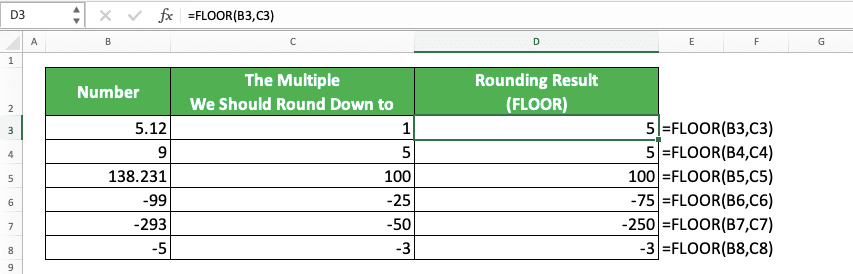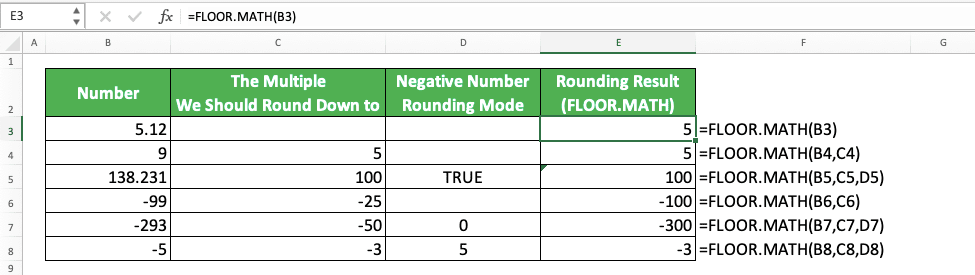You can see from the examples that FLOOR and FLOOR.MATH can round down positive numbers to the multiples we want. When you want to round down to the 1 multiple, then you don’t have to input any multiple in FLOOR.MATH.

You can see the differences in the negative number rounding process there too between FLOOR and FLOOR.MATH. FLOOR always rounds a negative number closer to zero while we can determine the rounding process using FLOOR.MATH.

If we don’t input anything for the rounding mode, then FLOOR.MATH will round a negative number away from zero. If we input any number other than zero there, then FLOOR.MATH will round a negative number close to zero.

Therefore, if you use FLOOR.MATH and want to round a negative number, choose the inputs you want to give correctly!

## How to Round Down Numbers to the Nearest Whole Number/Integer in Excel: INT

Need to round down a number with decimals to its nearest integer/whole number fast? There are few better options to do that in excel compared to using the INT formula.

The way to use INT in excel is very simple. You just need to input the number with decimals you want to round into INT like below.

= INT ( number_to_round_down_to_integer )

By using INT, you can get a whole number/integer from your decimal number fast! If you input a number with no decimals to INT, then this formula won’t do anything. Furthermore, if you input a negative number to INT, then it will round it to the nearest integer away from zero.

Here is the implementation example of INT in excel.As you can see in the example’s INT writings, we just need to input our decimal number to INT. Do that and we will immediately get the integer we want from that number!

## How to Remove Decimals in Excel: TRUNC

What if you want to just remove/truncate some decimals you have in your number? Excel also has a formula for that purpose. That formula is TRUNC.

Here is the general writing form of TRUNC in excel.

= TRUNC ( number_to_truncate_decimals_from , [ number_of_decimals_for_the_result ] )

The only input that you need to give to TRUNC is the number you want to remove decimals from. The input of the number of decimals for the result is optional. If you don’t input anything, then TRUNC will remove all the decimals in your number.

If you input a number with no decimals to TRUNC, then this formula won’t do anything to the number. If you input a negative number, then TRUNC will remove the decimals normally just as if you input a positive number.

For a better understanding of this formula, here is its implementation example in excel.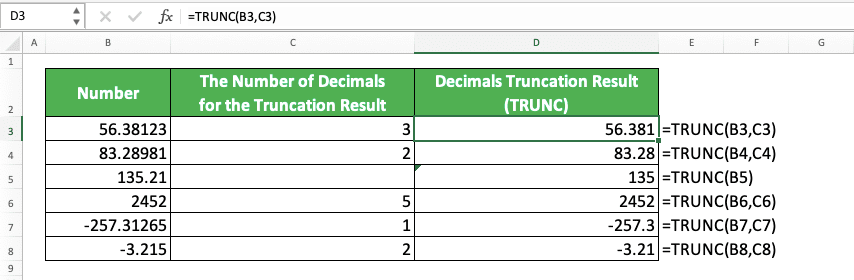The concept of using TRUNC is quite simple, isn’t it? If you need to truncate some or all of the decimals in your number, just use this formula!

## How to Round Up Numbers to the Nearest Even Whole Number/Integer in Excel: EVEN

Need to round up your decimal number to its nearest even whole number/integer? You can do that easily using EVEN.

Just like the INT that we discussed earlier, you just need to input the number you want to round to EVEN. Because of that, the EVEN formula writing form in excel becomes like this.

= EVEN ( number_to_round_up )

Simple, isn’t it? And here is its implementation example in excel.You can see in the example how EVEN rounds up a number into its nearest even integer. If the number is already an even integer, then EVEN won’t do anything. If the number is a negative number, then EVEN will round it to an even integer away from zero.

## How to Round Up Numbers to the Nearest Odd Whole Number/Integer in Excel: ODD

If what you need is to round your decimal to its nearest odd whole number/integer, then you can use ODD.

The way to write ODD is similar to EVEN. Just input the number you want to round up to its nearest odd integer to ODD.

= ODD ( number_to_round_up )

Here is its implementation example.You can see in the examples how ODD rounds up numbers to their nearest odd integers. Just like EVEN, if you input an odd integer to ODD, then it won’t do anything. If you input a negative number, then ODD will round it up away from zero to the nearest odd integer.

## How to Round Decimals Normally While Converting Them to Currency Format in Excel: DOLLAR

Need to round your decimal while also converting it to a dollar currency format? You can do that quickly in excel by using the DOLLAR formula.

The way to use DOLLAR is quite similar to ROUND. Here is its general formula writing form in excel.

= DOLLAR ( number_to_round , [ number_of_decimals_in_result ] )

In DOLLAR, you can give no input to the number of decimals you want in the rounding process result. If you do that, then DOLLAR will assume you want two decimals in the result. Just like what we often see in a standard dollar currency format.

If you give input to the number of decimals you want in the result, then it works just like ROUND. If you input the same or more number of decimals than your number currently has, then DOLLAR won’t round it (it will just add dollar currency format to your number).

If you input zero for the number of decimals, then it will round your number to an integer. If you input a negative number for that, then it will round your number to a powered 10 multiple. The power level of the 10 depends on the negative number value you give as input.

Here is the formula implementation example in excel.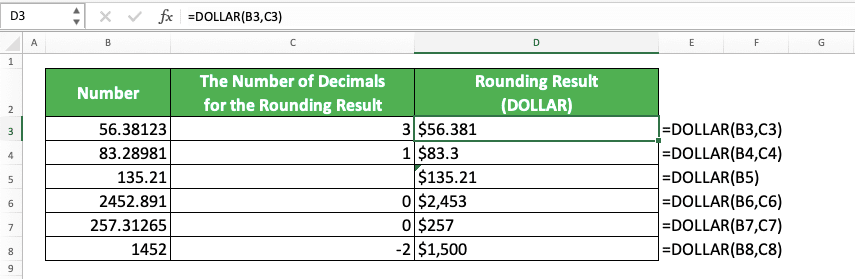Whenever you need the ROUND function while also converting your number to a dollar currency data format, just use DOLLAR!

## How to Round Decimals Normally While Converting Them to Formatted Number Text in Excel: FIXED

You can use FIXED if you want to round your number to a formatted number text. That means it will convert your number into text data format while adding a thousand delimiters (in commas or dots depending on your settings) to it if you want.

Here is how to write FIXED in excel generally.

= FIXED ( number_to_round , [ number_of_decimals_in_result ] , [ not_adding_thousand_delimiter_or_adding_it ] )

As you can see there, the only input you must give to FIXED is the number you want to round. The other two inputs, the number of decimals in the result and whether it shouldn’t add a thousand delimiters, are optional. If you don’t give inputs to both, then FIXED will round your number to two decimals and add a thousand delimiters.

For the input whether you want to add a thousand delimiters or not in the result, give TRUE or FALSE. TRUE means you don’t want to add them and FALSE means you want.

Here is the implementation example of FIXED in excel.As you can see, FIXED rounds our number into text and can add a thousand delimiters to the result. In the rounding result column, all the results align themselves left because they are in text data format (the default alignment of text in excel is left).

If you only need to change a number into a particular text format without rounding, then you can use TEXT too. TEXT offers more forms of text that you can apply to your number compared to FIXED.

## Example 1: How to Round a Number to 2 Decimal Places in Excel

Now that we have done discussing the rounding formulas in excel, it is time to take a look at some examples. These examples originate from the number rounding problems we sometimes face in excel.

An example of the problems is the rounding process to two decimal places. If we need to round a number with decimals, then we often need to round it to two decimal places. What are the excel rounding formulas we can use for that?

Well, from our list, there are definitely some formulas we can use like ROUND, ROUNDUP, ROUNDDOWN, and TRUNC. It all depends on the kind of number with two decimal places we want as a result.

Let’s implement some of those rounding formulas to this problem. In the screenshot below, we can see how we use ROUND, TRUNC, and FIXED to round some numbers to two decimals.As you can see in the example, we can use ROUND, TRUNC, and FIXED to round our numbers to two decimals. Just input two for the number of decimals you want in your rounding result in those formulas.

Whenever you need to round to two decimal places, just pick the right excel rounding formula for the result you want. Then, input two for the decimal places input in the formula you use!

## Example 2: How to Round a Negative Number in Excel

If you saw the examples when we discussed the excel rounding formulas, then you should see some negative numbers rounding examples. You can use excel formulas to round a negative number. However, each of the formulas has a different kind of treatment on negative numbers that you should pay attention to.

Some of them round your numbers away from zero while some round them closer to zero. Therefore, know the result you want and use the formula that can provide that kind of result for you. Check again the discussion of the formula you use in the previous parts of this tutorial if you need it.

For example, here are some negative numbers we round using ROUNDUP, ROUNDDOWN, and INT.As you can see in the example, each formula might give a different result when we round a negative number. ROUNDUP and INT will round it away from zero while ROUNDDOWN will round to closer to zero.

If you want to round a negative number normally, then you can use ROUND, DOLLAR, or FIXED instead.

## Example 3: How to Round a Number to the Nearest 0.5 in Excel

Need to round your number to the nearest 0.5 multiples in excel?

You can use multiple rounding formulas like MROUND, CEILING/CEILING.MATH, and FLOOR/FLOOR.MATH to do that. Just give 0.5 as the multiple input in the formula you use.

Here is an example of rounding a number to the nearest 0.5 using MROUND, CEILING, and FLOOR.You can see we get the 0.5 multiple rounding results by using those three formulas. Besides them, you can also use CEILING.MATH and FLOOR.MATH if their rounding process suits you.

One thing to note if you use MROUND to round a negative number to its nearest 0.5 multiple. You should add minus in front of your 0.5 input as not doing that will make MROUND produces a #NUM error.

## Example 4: How to Round a Number to the Nearest 5/10/50/100/500/1000 in Excel

Similar to rounding to the nearest 0.5 multiple, you should use MROUND, CEILING/CEILING.MATH, or FLOOR/FLOOR.MATH for this. Input the multiple you want to round to in the formula you use and you are good to go.

Alternatively, you can also use decimal rounding formulas like ROUND if you want to round to powered 10 multiples (10/100/1000/etc). Just input a negative number of decimals with the value depending on the power level of 10 multiple you want (-1 for 10, -2 for 100, -3 for 1000, etc). However, it might be simpler if you just use a multiple rounding formula like one of those five we mentioned before.

Here is the implementation example of multiple rounding formulas to round to the nearest 5/10/50/100/500/1000 multiple. We use MROUND, CEILING.MATH, and FLOOR.MATH here.As you can see in the example, you just need to input your number and multiple to the formula you use. Use MROUND if you want to round normally, CEILING/CEILING.MATH to round up, and FLOOR/FLOOR.MATH to round down.

## Example 5: How to Round a Percentage in Excel

If you want to round a percentage using an excel formula, remember that a percentage is equivalent to a decimal. For examples, 20% is actually 0.2 (20/100) and 5% is actually 0.05 (5/100).

Thus, you should consider the number of decimals that the percentage already has when you want to round. Add two to the number of decimals you input to your formula so it can produce the result you expect.

For example, here we round some percentages using ROUND, ROUNDUP, and ROUNDDOWN.In the examples, we want to round our percentage values to two, zero, and one decimal place. As you can see in the formula writings, we add two in the input of the number of decimals we want. As a percentage is a number we divide by 100, the two addition makes us get the correct results.

If you need to round the number of decimals look (not the real value) in your percentage, then use the increase decimal/decrease decimal feature. You can learn how to use the feature in the next part of this tutorial.

How about if we want to round our percentage value to a certain multiple of another percentage value? For this, you should use one of the excel multiple rounding formulas and input a percentage for the multiple input part.

Here is an example of that concept to make things clearer. We use MROUND, CEILING, and FLOOR to round our percentages to a multiple here.If you use a normal number as the multiple input, you won’t get the rounding result you want.

## Example 6: How to Round Time in Excel

What if, say, you want to round the time data that you have in excel? As the time rounding process is most likely a multiple rounding process, use MROUND, CEILING/CEILING.MATH, or FLOOR/FLOOR.MATH.

Just like a percentage multiple rounding, you should also use time as the multiple input in the formula you use. If you type your time data input to the formula directly, then you should use quotes (e.g. type “03:00:00” for three o’clock or three hours).

Here is an example of time rounding implementation in excel using MROUND, CEILING, and FLOOR.If you get a number result from your formula (that happens if your cell has a general data format), just change it into a time data format. To do that, highlight the cell where the result is, go to the Home tab, and click the data format dropdown. Choose Time from the dropdown list.## How to Round Numbers in Excel Without Formula 1: Increase Decimal/Decrease Decimal Menu

If you don’t want to round your number using a formula, then you can use some other features in excel. However, these features can only round the look of your number and not its real value. To round the value, you need to use an excel formula.

One of the features you can use for rounding numbers is the increase decimal and decrease decimal buttons. To use these buttons, highlight the cells that contain the numbers you want to round first.

Then, go to the Home tab and click the increase decimal button to add the number of decimals in your number. To reduce them instead, click the decrease decimal button.A pretty quick way to manage the number of decimals in your number, isn’t it? If you just want to adjust the look of the number of decimals in your number, use these buttons!

## How to Round Numbers in Excel Without Formula 2: Format Cell

You can also use the format cell menu to round the decimals look in your number.

Just right-click on the cell containing the number you want to round and choose Format Cells....In the dialog box that shows up, go to the Number tab and click the Number category there. On the right, you will find a Decimal Places text box. In there, you can enter the number of decimals you want for your number.After you finish entering the number of decimals you want, click OK.Your number will immediately have the number of decimals you specify in the text box!## How to Round Numbers in Excel Without Formula 3: Custom Format

Besides using the Number data format in the Format Cells menu, you can also use Custom one to round your numbers.

For this, in the Format Cells dialog box’s Number tab, click the Custom category. In the Type: text box, type zero (represents your whole number), your decimal delimiter (dot or comma depending on your computer settings), and zeroes which represent the number of decimals you want.In the example above, three zeroes behind the dot means we want to round our number to three decimals.

After you finish typing your number format, click OK.Your number will immediately have the number of decimals you specify in the Custom data format text box!## Exercise

After you have learned how to round numbers in excel using various formulas and features, now let’s do an exercise. You should do it so you can deepen your understanding on what you just learned from this tutorial.

### Questions

1. What is the rounding result if we should round down the number to 2 decimals?
2. What is the rounding result if we should round the number to the nearest 3 multiple?
3. What is the rounding result if we round the number to a whole number using TRUNC?

TRUNC with no number of decimals input functions quite similarly to INT. However, if you round a negative number, then TRUNC will round it closer to zero (because it just truncate all the decimals). INT, meanwhile, will round it away from zero.

Related tutorials you should learn:

Get updated excel info from Compute Expert by registering your email. It's free!